Study Guide

# Electromagnetism Questions

## Electromagnetism Questions

Question 1. Using Gauss’ Law, derive the electric field within a uniformly charged solid sphere of total charge Q where R is the radius of the sphere itself and r is any distance from the center out to that radius, such that 0 ≤ rR.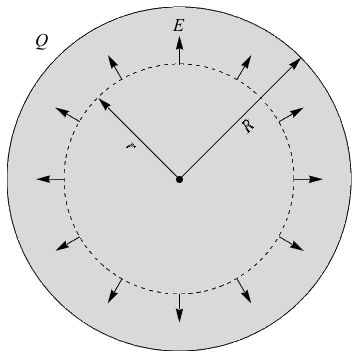Answer: Gauss’ Law being about flux like it is, let’s pull out the corresponding equation of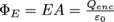. The enclosed charge for some radius r corresponds to the ratio of the volumes of the entire sphere of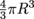to the smaller sphere of volume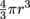within, leading to the ratio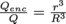.

Solving for Qenc and then substituting it into Gauss’ Law creates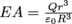. The surface area of a sphere of radius r is 4πr2, which allows us to solve for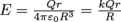. Nicely done.

Question 2. The current density J is written as J = σE. Relate Ohm’s law to this expression by defining the inverse of conductivity σ as ρ. Hint: resistance at a microscopic level has units of Ωm.

Answer: Ohm’s law states that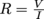, where R is resistance, V is the potential difference and I is the current.

With our new definition for current density, we write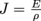. If the current has a uniform density, then I = JA, where A is the cross-sectional area.

Using, we then write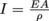.

Now let’s say the conductor has length l with a potential difference of V = El. Going back to Ohm’s law we have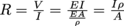.

We started this problem defining ρ as the inverse of conductivity, i.e.resistance. The units we’re left with are Ω m. Bingo!

Question 3. Show that a change in an electron’s orbital speed is equivalent to a change in magnetic dipole moment. This is the basis behind diagmagnetism. Assume the electron is revolving around the nucleus in a circle of radius R (hopefully the quantum gods show mercy for this simplification) with a short enough period T so that the current is steady enough.

Answer: Two things to remember right off the bat. Number one, the magnetic dipole of a current loop is given by m = IA and Number two, the period of a body undergoing circular motion is given by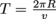, where v is the orbital speed.

Let’s set up our problem such that our little electron buddy circles the z-axis as seen below: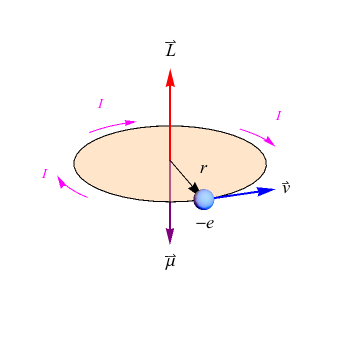So, what’s current equal to again? All together now: charge over time. In this case,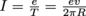, where e is the elementary charge, the value of the charge of a single proton or electron. We’ll be leaving this quantity in terms of e, but for the record, its value is 1.6 × 10-19 C.

Now, if we remember, a loop of conducting wire (or the electron circling the nucleus in this case) will experience a torque of magnitude τ = IBAsin ø, where m = IA and is the magnetic dipole moment. For us, sin ϕ = 1. Together with our previous calculation, we write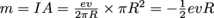in the z-direction.

What’s next? The question asks us to show that a change in orbital speed will give us a change in the magnetic moment. Here’s something to realize: depending on the magnetic field’s orientation, the electron will either slow down or speed up from speed v to speed v’, since it will be subjected to the Lorentz force on top of the Coulomb force. With our centripetal acceleration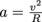, we’re not just dealing with an electric force alone anymore. We’ve got a magnetic one too. Instead of writing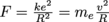, where me is the mass of the electron, we need to write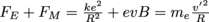, where v’ is the new speed of the electron with this additional magnetic force. We assume the magnetic field is perpendicular to the plane of the electron’s orbit and pointing upwards, so that v’ > v).

So far so good? Great. Then let’s carry on.

We rewrite our equation as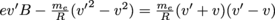. Now, if we let Δv = v’- v and we assume Δv is extremely small, then we can rewrite everything as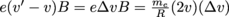, such that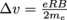. Going back to our magnetic dipole equation, m = –½evR in the z-direction, we can then write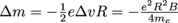.

Question 4. A solenoid consists of a long straight coil of wire wrapped around a bar magnet. Derive the magnetic field B of the solenoid using Ampere’s law if it has n turns per unit length, carries current I, and hasa circular cross section of radius R.

Answer: To find the magnetic field, we need to use Ampere’s law, Bd = μ0I..
Let’s draw a rectangular amperian loop of length l over the solenoid: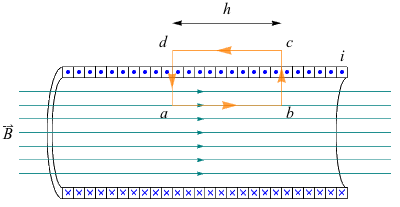Here you can see the current flowing through the solenoid coils as they go in and out the page, in on x’s and out on dots. The magnetic field B points towards the right.

Outside the solenoid the magnetic field is zero, so the top portion of the amperian loop doesn’t contribute to the current. What about the two vertical sides? They’re at right angles to the magnetic field, so their contribution is also zero. We’re only left with the bottom of the loop with length l.

B is constant, and the solenoid has n turns per unit length l. This means the amperian rectangular loop encircles nl turns. Since the same current I flows through each turn, we get that Iencircled = nlI. This gives us Bl = µ0nlI, or B = µ0nI.

Question 5. Find the magnetic flux ΦB through one single turn of the solenoid in #4.

Answer: We know that magnetic flux is given by the equation that ΦB = BA. The magnetic field in a solenoid is given by B = µ0nI. The solenoid has a circular cross section of radius R, so its area is A = πR2. That gives us a magnetic flux through a solitary loop of wire of ΦB = µ0nIπR2. Isn’t that the same as for all the loops? It should be: the magnetic field in a solenoid is constant.

Question 6. After many months of persuasion, we convince Master Yoda to train us as Jedis on his home planet Degobah. To demonstrate the power of the Force, Yoda levitates a conducting loop of wire of mass m = 1 kg, resistance R = 2 Ω, and radius r = 1.5 m at a height of 10 m from the ground to the center of the loop.

As a young Jedi apprentice, your first task consists of creating a uniform magnetic field that increases at a rate of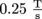. The only tools at your disposal are the Force, which is equivalent to how quickly we can move electrons along the wire, and the levitated loop of wire with the physical properties mentioned above. Per Yoda’s instructions, the magnetic field can either point downwards or upwards, as long as it intersects the loop of wire at a perpendicular angle. Yoda is kind enough to assist to ensure the wire remains levitated so that we may focus on creating a uniform magnetic field. How much Force must we conjure up to perform this task?

Answer: The first part of this problem involves taking a deep breath and repeating the first line of The Hitchhiker’s Guide to the Galaxy: DON’T PANIC.

Unfortunately, the answer is not 42.

The key to solving this problem lays in the concept of “the Force”, which is defined as the ability to quickly move electrons along the wire, i.e.create a current.

The magnetic flux of is given by: Φ = BA where B is the magnetic field we’re supposed to create and A is the area of the loop, πr2 in this case, so Φ = πr2B.

We don’t care one iota about the strength of the magnetic field itself. What matters is the change of flux for Faraday’s Law. Hence the magnitude of the EMF ε is given by the change of flux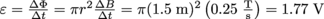.

Using Ohm’s Law, we can then calculate the Force (current) needed with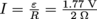, which is 0.88 A.

Question 7. Unfortunately, since Luke was hanging out last night with Han Solo at the Cantina, it takes him three tries to accomplish the simple task from #6. We did it, but not Luke. Yoda is not a happy camper (happy camper he is not). He morphs the loop of wire into a perfect square, places it such that half of one of its side intersects parallel to Luke’s crafted magnetic field, and orders him to use his disappointing abilities to move the entire square loop of wire out of the magnetic field in exactly 10 seconds. Since he’s feeling rather greenish, Yoda cuts him some slack and tells Luke the magnetic field can stop increasing since it requires so much Force on his part. The magneticfield returns to its original value of 5 T. How much Force must Luke use to accomplish this new task, if the Force is once again represented by current flow?

Answer: Again, we don’t care a jot about the flux. What matters is how quickly the flux is changing in the area of the loop exposed to the now-constant magnetic field.

Once again, Φ = BA, except that this time, the area of the conducting loop is changing. The area of the square exposed to the magneticfield is simply the length l of the wire multiplied by whatever portion of the square is exposed to the magnetic field. If we define this portion of the square to be x, we find that the area is equal to A = lx.

So using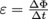, we have that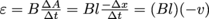, where v is the velocity of the square of wire, decreasing according to Lenz’ Law. Think about it: the induced current created a new magnetic field that opposes the change in magnetic flux, so the square loop slows down.

To find the new area, we need to remember that the poor conducting wire was forced to undergo metamorphosis, going from the shape of a happy circle to a sharp square at a snap of Yoda’s fingers. Its radius was 1.5 m, which means its circumference was C = 2πr = 9.42 m. The circumference is equal to the total perimeter.. This means the square has 4 equal sides that measure 2.36 m each. If half of a side of one of the squares crosses the magnetic field in 10 seconds, then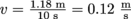, which means that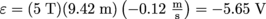. Finally, using Ohm’s Law,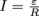, which gives a current of -2.83 A.

Congratulations, Luke! Now you’re a Jedi!

Question 8. A positively charged particle is positioned above a current-carrying wire that has an equal number of positive and negative charges. What forces (magnitude and direction) does the particle feel when it’s at rest?

Answer: Since the current is carrying equal positive and negative charges, the wire is neutral, so it doesn’t produce an electric field. Exactly how it does carryies a neutral current, we’re not sure. This is all hypothetical. Since the charges are moving, however, they produce a magnetic field B. The particle isn’t moving though, so it doesn’t experience a magnetic force anyway. A charged particle rest experiences zero magnetic force, and there’s no electric force for it to experience for a net force of zero.

Question 9. What forces does the particle in #8 experience when moving to the right with velocity u?

Answer: Now the particle begins to move to the right with a velocity u relative to the wire. A picture is always worth a thousand words. Let’s take a look at the following diagram: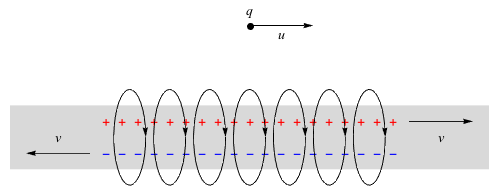A charge q positioned at a distance r from a current-carrying wire with magnetic field B on the charge pointing out of the page.

Since the particle is moving, it experiences a Lorentz force equal to F = qu × B. With the right-hand rule, we can see that this force is pointed towards the wire.

Question 10. In our relativistic electrodynamics lens, we talked about how relativistic corrections weren’t needed for the subject of electromagnetism. This means that studying the above scenario from any frame of reference should in theory be equivalent. What forces are at play according to a) the wire and b) the charge’s frame of reference?

Answer: The frame of reference of the wire has already more or less been looked at already. It produces no electric field but its current forms a magnetic field, which loops around its length. If a moving particle goes to the right, it experiences a magnetic force F, as we’ve talked about.

The particle’s frame of reference, on the other hand, presents a more interesting case. It’s moving to the right. You can also check out the positive charges in the wire moving to the right, while the negative ones are moving to the left. What does it mean for our particle?

Simply stated, that positive charges in the wire have a smaller speed than their negative counterparts according to relativistic velocity addition. According to our heroic particle, which is endangering its life in order for us to understand relativistic electrodynamics, the negative charges are moving a lot faster now, with a magnitude of v + u. The distance between two negative charges is contracted more according to the particle (and to the laws of special relativity) than the distance between the positive charges.

But hang on a second. Charge is invariant. This means charge density (charge per unit length) gets larger for the negative charges and smaller for the positive charges. What are we left with?

That’s right– an overall negative charge density according to the particle. All because it happens to be moving. Kinda crazy, right?

Now that we have a negative charge on the wire, it produces an electric field, to which the positively charged particle is attracted. Yes, the wire’s magnetic field is still there, but since the particle is at rest in its own frame of reference, it’s irrelevant. Rather, the particle is attracted to the wire because of the Coulomb force, not the Lorentz force.

And there we have it: the same result explained by two different phenomena: electric and magnetic, bridged together by the genius of relativity.